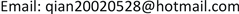1. 引言

2. 深度神经网络

3. 深度学习提取色彩特征方法

L content ( p → , x → , l ) = 1 2 ∑ i , j ( F i j l − P i j l ) (1)

∂ L content ∂ F i j l = { ( F l − P l ) i , j         F i j l > 0 0                                       F i j l < 0 (2)

G i j l = ∑ k F i k l F i k l (3)

L style ( a → , x → ) = ∑ l = 0 L w l E l (5)

∂ E l ∂ F i j l = { 1 N l 2 M l 2 ( ( F l ) T ( G l − A l ) ) j i           F i j l > 0 0                                                                                 F i j l < 0 (6)

4. 唐卡风格特征迁移

5. 结论